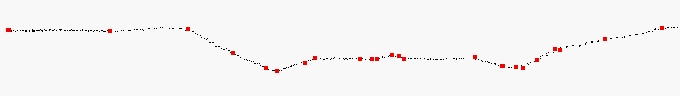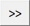# TerraScan User Guide

 Navigation: Batch Processing Reference > Classification Routines > Points Model keypoints

Model keypoints

Model keypoints routine classifies points which are needed to create a triangulated surface model of a given accuracy. This routine is normally used to create a thinned data set from previously classified as ground points. For LAS 1.4 files, you can set the keypoint bit instead of classifying the points.

The routine tries to find a relatively small set of points (= keypoints) which create a triangulated model of the given accuracy. It starts by classifying all points from the source class, which is usually the ground class, into the target class. Then, it iteratively removes all points from the target class that are not required in a surface model of the given accuracy.Model keypoints (red points) as subset of ground points (black points).

You control the accuracy of the keypoint model with elevation tolerance settings Above and Below model. These settings determine the maximum allowed elevation difference between the keypoint model and a surface model created from the original ground points. The Use points every setting ensures a minimum point density in the final model. For example, if the distance between a point and its closest neighbour must not be less than 10 meter, you should set the Use points every setting to 10.0 m.SETTING

EFFECT

From class

Source class(es). Contains usually ground points.Opens the Select classes dialog which contains the list of active classes in TerraScan. You can select multiple source classes from the list that are then used in the From class field.

To class

Target class. For LAS 1.4 files, you can choose Set keypoint bit as alternative.

Inside fence only

If on, points inside a fence or selected polygon(s) are classified.

Use points every

Minimum point density of model keypoints. In areas of completely flat ground, there is a keypoint at approximately the given distance.

Tolerance above

Maximum allowed elevation difference upward between the triangulated keypoint model and a model of the original source points.

Tolerance below

Maximum allowed elevation difference downward between the triangulated keypoint model and a model of the original source points.

TerraScan User Guide   26.07.2021   © 2021 Terrasolid Ltd